2016年05月15日

# CNN反向求导及推导

$Loss=-\frac{1}{m}\sum_{i=1}^{m}y_{i}{}'logf\left ( x_{i} \right )+\lambda\sum_{k=1}^{L}sum\left ( \left \|W_{k} \right \|^{2} \right )$

## 问题一：求输出层的误差敏感项。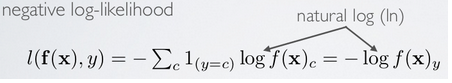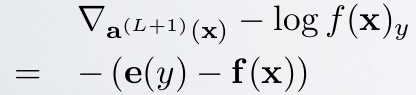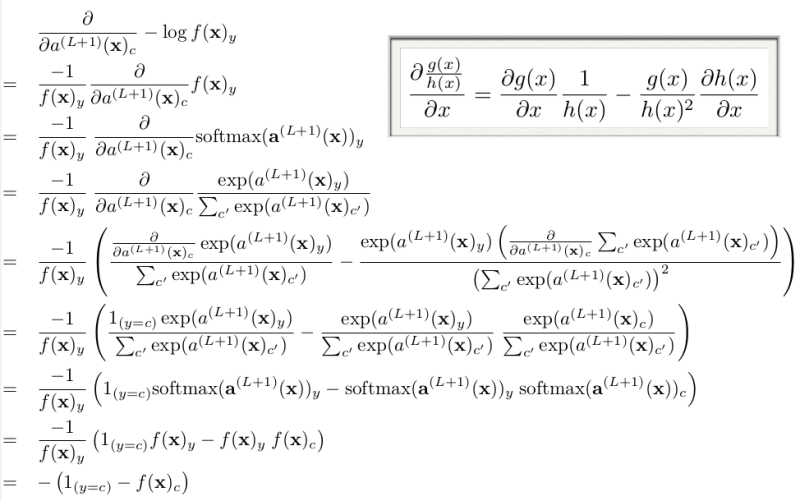## 问题二：当接在卷积层的下一层为pooling层时，求卷积层的误差敏感项。

$\delta _{j}^{l+1}$

$\delta _{j}^{l}$$h\left ( a_{j}^{l} \right ){}'$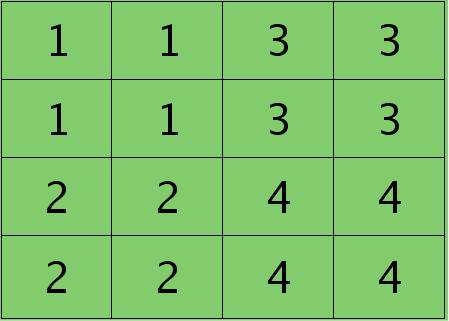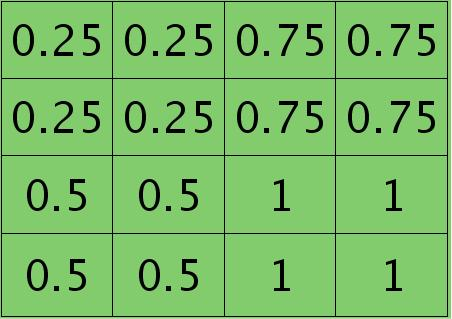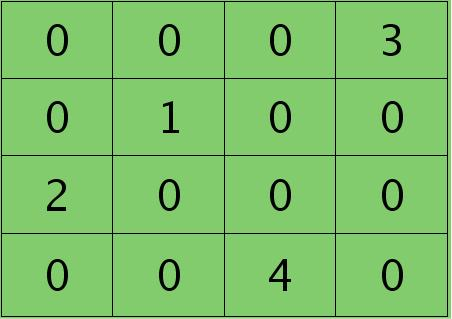## 问题三：当接在pooling层的下一层为卷积层时，求该pooling层的误差敏感项。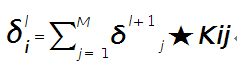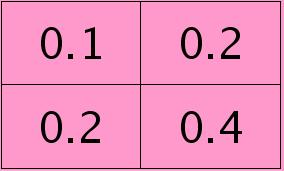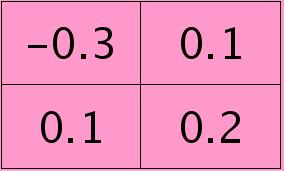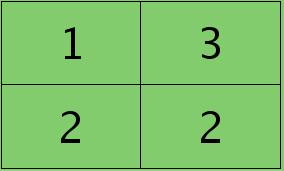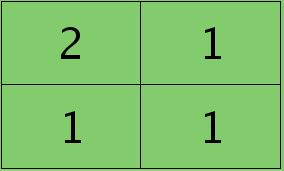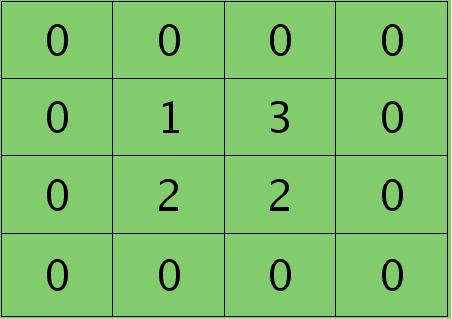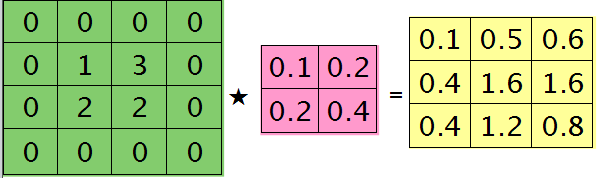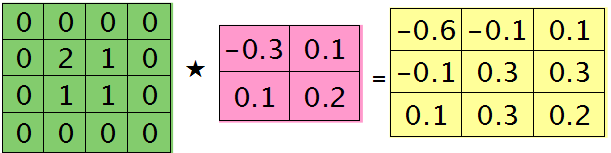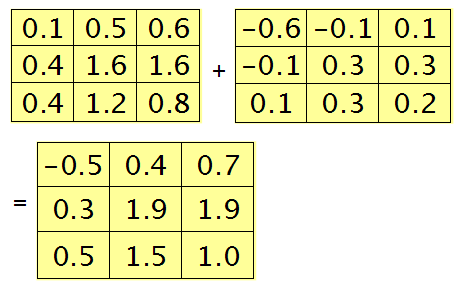## 问题四：求与卷积层相连那层的权值、偏置值导数。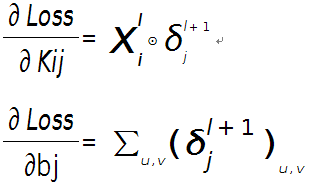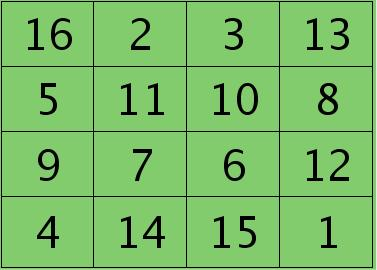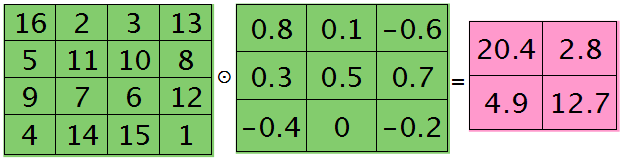## 总结

### 当前层的滤波器$\delta$更新等于前一层的feature map的值和当前层feature map的$\delta$值的相关操作。blog comments powered by Disqus